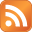# How to create a visual status progress bar in a Google Sheets spreadsheet

Published on April 21st 2020 - Listed in Internet Cloud Personal

When going into "project mode", it is always nice to have a visual representation of the current status. Whether this status is a single task or the whole project, it helps to quickly interpret how far we've come and how long the remaining road still is.

## Creating a progress bar using sparkline

In Google Sheets, there is a nice way how to create such a simple "progress bar", using the SPARKLINE function. This function uses a value of a field and fills the field with a color - depending on the amount of the selected value and how this value is represented in between given min and max values.

Sounds complicated when writing it down, but by looking at a practical example this turns out to be not complicated at all:

In this example the selected field (C3) used to represent the task progress. It uses the following formula:

=SPARKLINE(B3,{"charttype","bar";"max",100;"min",0;"color1","green"})

B3 in this case is the value which SPARKLINE should use. The value of this field is set to 100, which represents the task completeness in percent. 100% of course means that this task was finished. SPARKLINE now compares this value (100) with the min and max values in its function settings. As the value (100) is the same as its defined max (100), the field C3 is fully field with the progress bar.

Further down in row 9 another example shows up, where the task "Base setup" was completed at 50% (value of B9). The same formula (adjusted for the field value of course) automatically creates a progress bar filling up exactly half of the field C9.

Note: The idea to use SPARKLINE was found on a Reddit post. Actually the very first time I found something useful on Reddit... ;-)

## What about progress bar of the whole project?

The SPARKLINE function can also be used to show a progress bar of all tasks combined. As the example above shows, once the percentage of a task is known, it's pretty easy to create the progress bar. Speaking in terms of the whole project the big question is: How are all tasks calculated together?

A simple way (without setting weights on the tasks) is to create a SUM of all percentage values and divide the sum by the number of fields. In order to automatically get the number of fields, the function COUNTA can be used. This counts the numbers of fields which contain a value; empty fields (in this case category rows 2, 6, 8 and 12) are ignored.

=SUM(B2:B14)/COUNTA(B2:B14)

This results in an average percentage:

Now that the project has a (again, very simplified!) overall progress percentage, this value can be used to create a status progress bar across the whole project (here with a red color):

## The final mile: Project progress with task weighting

The project progress in the above example shows some representation of the project. But the representation is only correct if all tasks use the same resources, for example the same amount of time to complete.

In order to fine-tune the whole project progress calculation, a "weight" value per task is needed. In the example below, the "Time" column is used to specify a weight on each task. Or explained differently: How time consuming is each task?

By using the SUMPRODUCT formula, values of a range (B2:B14) can be multiplied with corresponding values of another range (C2:C14). The sum of all of these multiplications (hence the name SUMPRODUCT) can be divided by the sum of the weight (total time) prepared in B16:

=(SUMPRODUCT(B2:B14,C2:C14))/B16

The full project status is now at 75% - much higher than the previous 55% without task weights. This is a much more realistic project progress representation as the tasks more time consuming are weighing higher on the calculation.